# Wheatstone bridge

A Wheatstone bridge is a circuit that is used to measure an unknown resistance. This is done by balancing two legs of a bridge circuit. One of which includes the unknown resistance. The Wheat stone consists of four resistors connected as a square. The circuit is balanced when R 2 /R 1 = R x /R 3 . The current will be zero in this case. If the circuit is not balanced, a current will flow and can then be measured.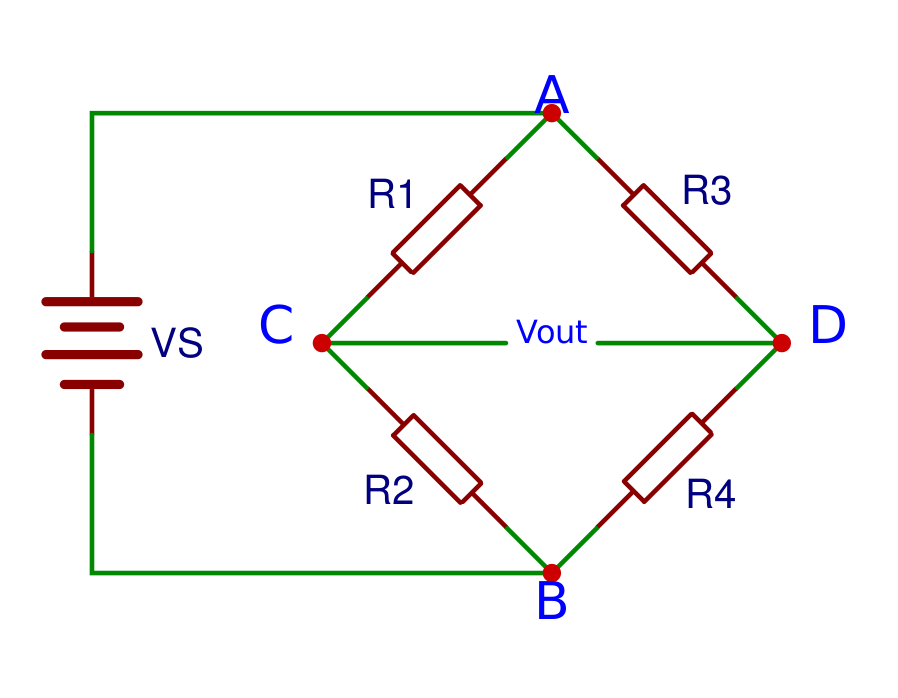### Formulas

When the Wheatstone bridge is balanced, The unknown resistance can be calculated with one of the following formulas: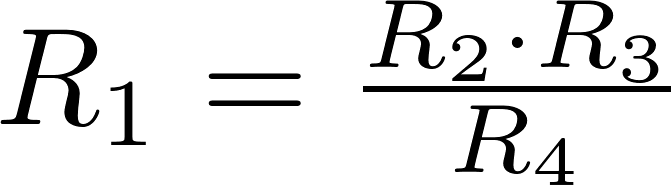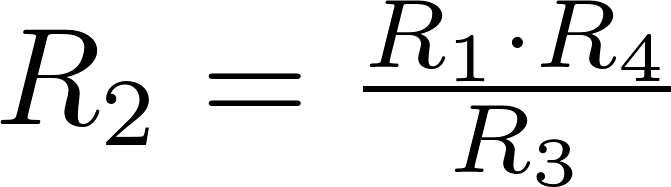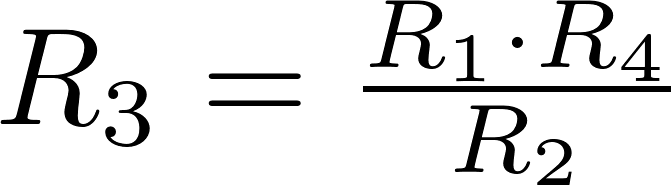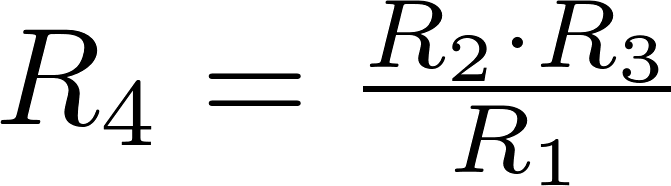The output voltage can be calculated with the following formula if all resistances and the input voltage are known: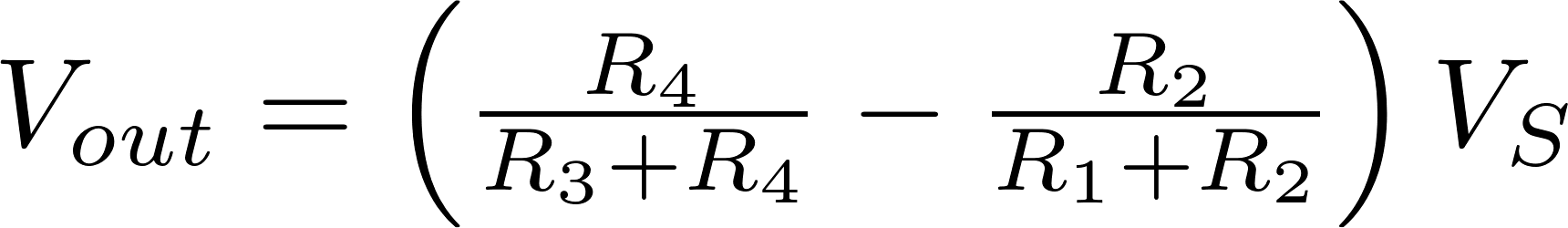R is the symbol for resistance and is measured in ohm (Ω).
V is the symbol for voltage and is measured in volt (V).
V out is the output voltage and is measured in volt (V)

### Calculators

#### Resistance

You can use this calculator to calculate an unknown resistance.

#### Output voltage

You can use this calculator to calculate the output voltage by entering all resistances and the input voltage.